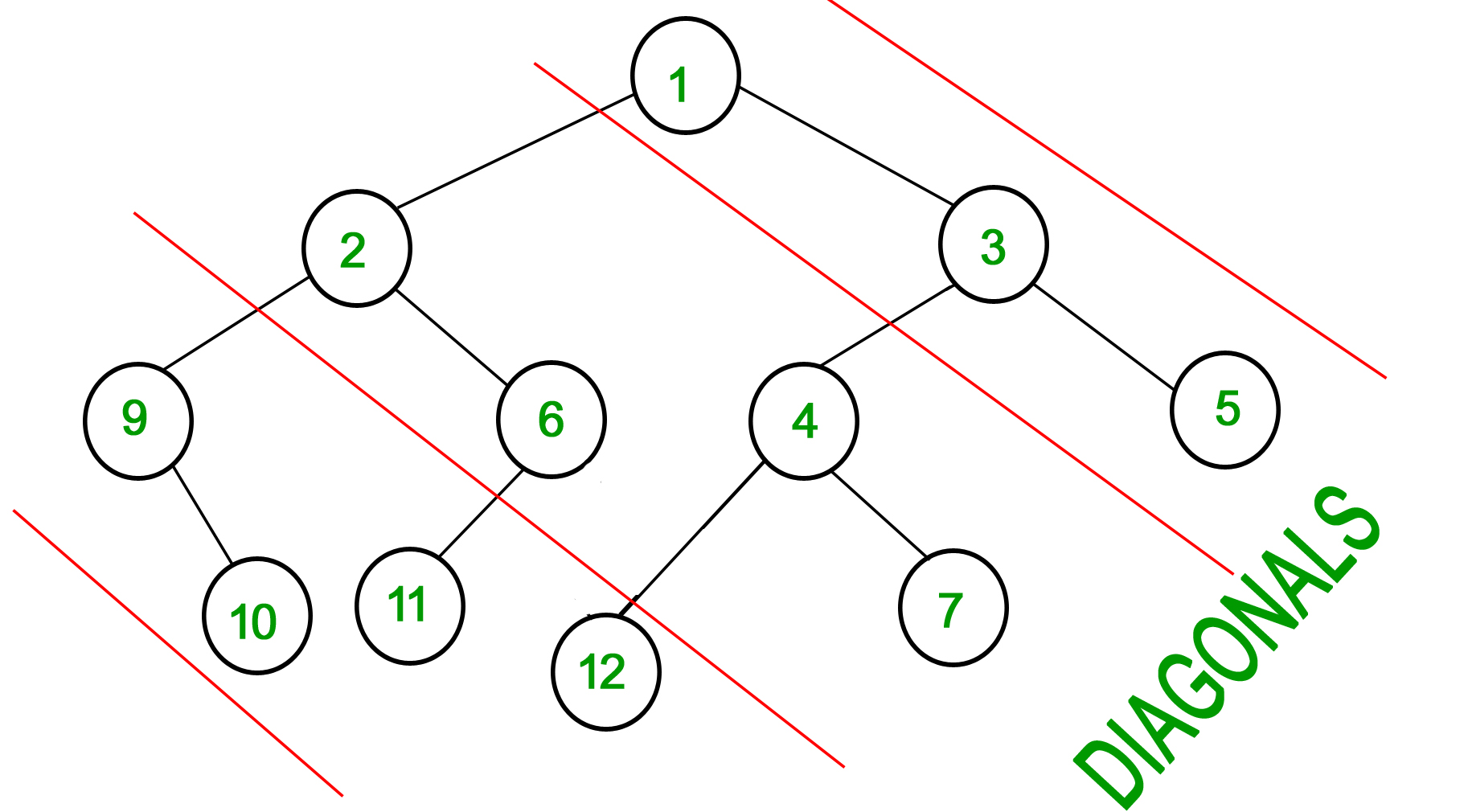Diagonal Sum In Binary Tree
Easy Accuracy: 51.18% Submissions: 15853 Points: 2

Consider Red lines of slope -1 passing between nodes (in following diagram). The diagonal sum in a binary tree is the sum of all node’s data lying between these lines. Given a Binary Tree of size N, print all diagonal sums.

For the following input tree, output should be 9, 19, 42.
9 is sum of 1, 3 and 5.
19 is sum of 2, 6, 4 and 7.
42 is sum of 9, 10, 11 and 12.Example 1:

Input:
4
/   \
1     3
/
3
Output: 7 4


Example 2:

Input:
10
/    \
8      2
/ \    /
3   5  2
Output: 12 15 3


You don't need to take input. Just complete the function diagonalSum() that takes root node of the tree as parameter and returns an array containing the diagonal sums for every diagonal present in the tree with slope -1.

Expected Time Complexity: O(N).
Expected Auxiliary Space: O(N).

Constraints:
1<=Number of nodes<=105

Note: The Input/Ouput format and Example given are used for system's internal purpose, and should be used by a user for Expected Output only. As it is a function problem, hence a user should not read any input from stdin/console. The task is to complete the function specified, and not to write the full code.

We are replacing the old Disqus forum with the new Discussions section given below.

### Editorial

We strongly recommend solving this problem on your own before viewing its editorial. Do you still want to view the editorial?

#### My Submissions: